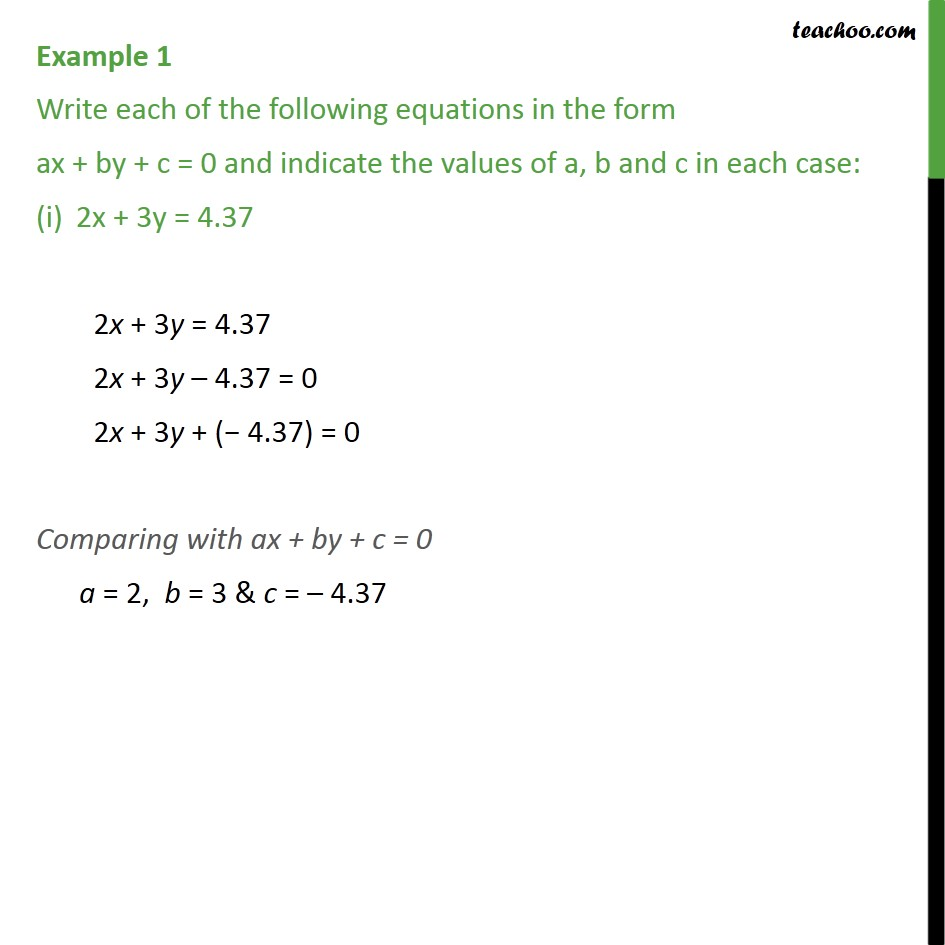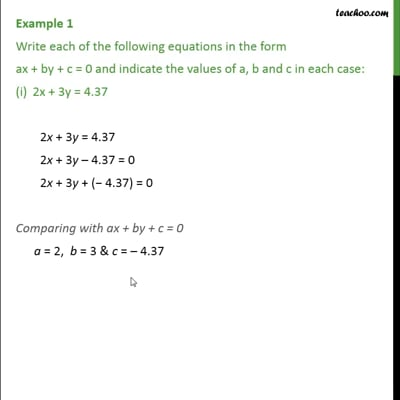Definition and General Form of Linear EquationThis video is only available for Teachoo black users

Solve all your doubts with Teachoo Black (new monthly pack available now!)

### Transcript

Example 1 Write each of the following equations in the form ax + by + c = 0 and indicate the values of a, b and c in each case: 2x + 3y = 4.37 2x + 3y = 4.37 2x + 3y – 4.37 = 0 2x + 3y + (− 4.37) = 0 Comparing with ax + by + c = 0 a = 2, b = 3 & c = – 4.37